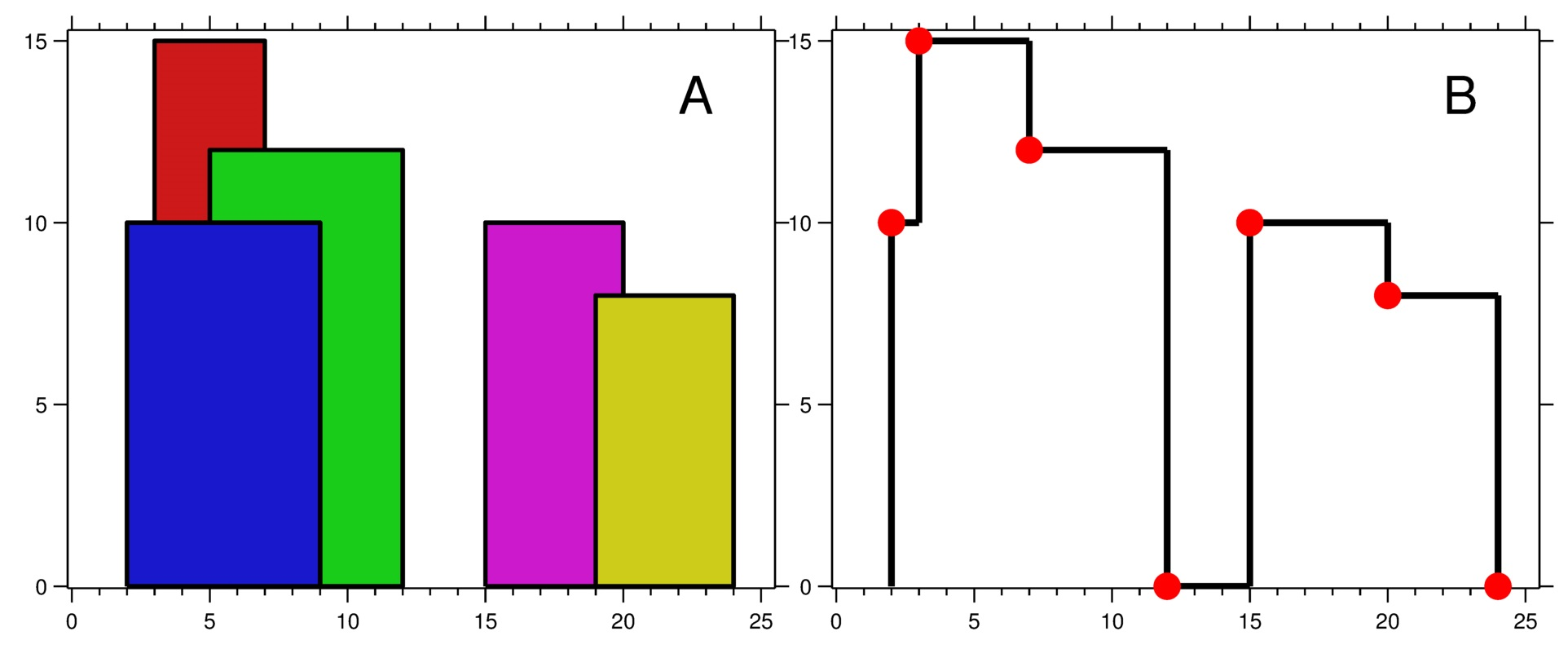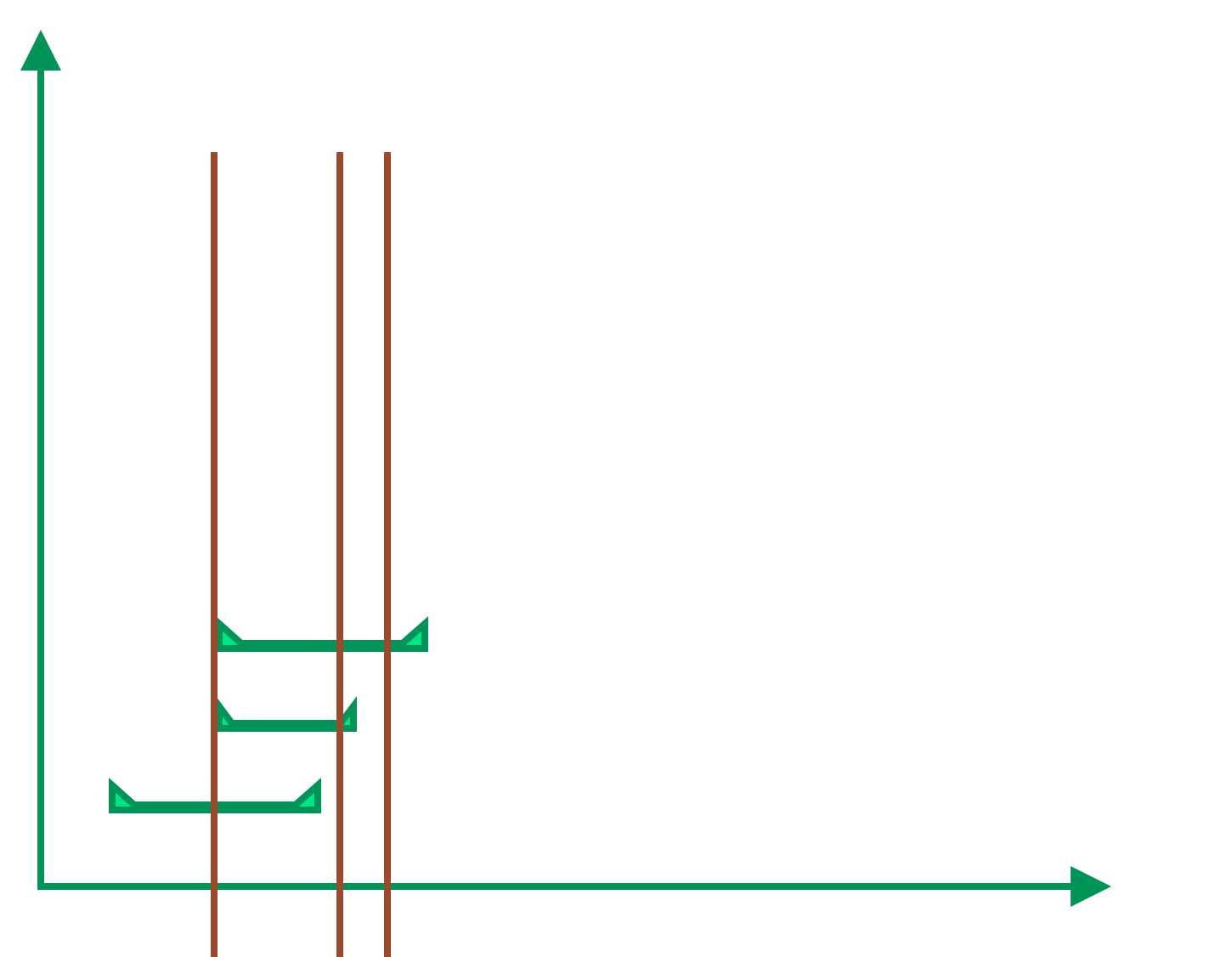# 扫描线算法

05 May 2021

#### 定义#### 一般解题步骤

1. 数据排序
2. 扫描排序后的数据，聚合有共性的数据
3. 用一个新的数据结构存聚合后的结果

#### 天际线问题

• `lefti` 是第 `i` 座建筑物左边缘的 `x` 坐标。
• `righti` 是第 `i` 座建筑物右边缘的 `x` 坐标。
• `heighti` 是第 `i` 座建筑物的高度。```输入：buildings = [[2,9,10],[3,7,15],[5,12,12],[15,20,10],[19,24,8]]

```输入：buildings = [[0,2,3],[2,5,3]]

```

• `1 <= buildings.length <= 104`
• `0 <= lefti < righti <= 231 - 1`
• `1 <= heighti <= 231 - 1`
• `buildings``lefti` 非递减排序

1. `int[n] buildings` 重构成一个`List<int>` 的数组all，`int` 0 位表示x坐标，1位表示y坐标，左边转成负数，然后可以按照x从小到大排序，x相等则y从小到大排序(左边的y是负数，所以绝对值是按从大到小排序)
2. 使用一个优先队列来缓存当前扫描到的位置所有建筑的高度
3. 遍历all中的元素，遇到y为负数是建筑的起始位置，加入优先队列，遇到y为正数是建筑的结束位置，从优先队列中移除这个高度；每遇到一个新的点都要判断是否为新的高度，如果是加入结果集
``````// java
class Solution {
public List<List<Integer>> getSkyline(int[][] buildings) {
List<int[]> all = new ArrayList<>();
for (int[] e : buildings) {
all.add(new int[]{e, -e}); //left top corner
all.add(new int[]{e, e}); //right top corner
}
// sort by x asc, if x is equal , by y desc
all.sort((o1, o2) -> o1-o2==0 ?  o1-o2 : o1-o2);

List<List<Integer>> res = new ArrayList<>();
// queue to store heights
PriorityQueue<Integer> heights = new PriorityQueue<>(Comparator.reverseOrder());
// when the last build gone, the max height is 0

// last point's height
int maxHeight = 0;
for (int[] p: all) {
// p < 0 left corner, add height to stack
// else right corner, remove height from stack
else heights.remove(p);

// meet change point, add it to the result list
if (maxHeight != heights.peek()) {
maxHeight = heights.peek();
}
}

return res;
}
}
``````

#### 包含每个查询的最小区间

```输入：intervals = [[1,4],[2,4],[3,6],[4,4]], queries = [2,3,4,5]

- Query = 2 ：区间 [2,4] 是包含 2 的最小区间，答案为 4 - 2 + 1 = 3 。
- Query = 3 ：区间 [2,4] 是包含 3 的最小区间，答案为 4 - 2 + 1 = 3 。
- Query = 4 ：区间 [4,4] 是包含 4 的最小区间，答案为 4 - 4 + 1 = 1 。
- Query = 5 ：区间 [3,6] 是包含 5 的最小区间，答案为 6 - 3 + 1 = 4 。
```

```输入：intervals = [[2,3],[2,5],[1,8],[20,25]], queries = [2,19,5,22]

- Query = 2 ：区间 [2,3] 是包含 2 的最小区间，答案为 3 - 2 + 1 = 2 。
- Query = 19：不存在包含 19 的区间，答案为 -1 。
- Query = 5 ：区间 [2,5] 是包含 5 的最小区间，答案为 5 - 2 + 1 = 4 。
- Query = 22：区间 [20,25] 是包含 22 的最小区间，答案为 25 - 20 + 1 = 6 。
```

• `1 <= intervals.length <= 105`
• `1 <= queries.length <= 105`
• `queries[i].length == 2`
• `1 <= lefti <= righti <= 107`
• `1 <= queries[j] <= 107```````class Event implements Comparable<Event> {
// 0 -> left point; 1 -> query; 2 -> right point
int type;
// position
int pos;
// left point, right point -> interval; query -> index
int para;

public Event(int type, int pos, int para) {
this.type = type;
this.pos = pos;
this.para = para;
}

@Override
public int compareTo(Event that) {
// 按x在位置排序，如果x相同，则左端点=》查询事件=》右端点
return this.pos==that.pos ? this.type-that.type : this.pos-that.pos;
}
}
``````

`````` public int[] minInterval(int[][] intervals, int[] queries) {
/**
* 方法一：离线算法, 扫描线算法
* 提示1
* 如果我们可以将给定的区间和询问重新排序，那么是否可以使得问题更加容易解决？
*
* 提示2
* 我们可以将区间的左右端点以及询问都看成一个「事件」，如果我们将这些「事件」按照位置进行升序排序，那么：
* 如果我们遇到一个表示区间左端点的「事件」，那么可以将该区间加入某一「数据结构」中；
* 如果我们遇到一个表示区间右端点的「事件」，那么可以将该区间从「数据结构」中移除；
* 如果我们遇到一个表示询问的「事件」，那么需要在「数据结构」中寻找最短的区间。
* 提示3
* 你能想出一种合适的「数据结构」吗？
*/

class Event implements Comparable<Event> {
// 0 -> left point; 1 -> query; 2 -> right point
int type;
// position
int pos;
// left point, right point -> interval; query -> index
int para;

public Event(int type, int pos, int para) {
this.type = type;
this.pos = pos;
this.para = para;
}

@Override
public int compareTo(Event that) {
return this.pos==that.pos ? this.type-that.type : this.pos-that.pos;
}
}

List<Event> eventList = new ArrayList<>();
for (int i=0; i<queries.length; i++) {
}

for (int i=0; i<intervals.length; i++) {
int interv = intervals[i] - intervals[i] + 1;
// left point
// right point
}

eventList.sort(Event::compareTo);

int[] ans = new int[queries.length];
PriorityQueue<Integer> seq = new PriorityQueue<>();
for (Event e: eventList) {
switch (e.type) {
case 0: seq.add(e.para); break; //left point
case 1: ans[e.para] = seq.isEmpty() ? -1
: seq.peek();
break; //query
case 2: seq.remove(e.para); break; //left point
}
}
return ans;
}
``````

1. 将间隔`intervals` 按照左端点从小到大排序
2. 因为结果需要按照 `query` 的顺序，所以将`query` 转化成 `int[]` 的数据结构，0位存query的值，1位存query的序号，然后按query的值从小到大排序
3. 遍历`query`的值，按顺序查询排序后的`interval`，并用一个优先队列缓存满足条件的`intervals`
``````// 双排序队列，滑动查询，优先队列
public int[] minInterval(int[][] intervals, int[] queries) {
// 区间按照开始位置排序
Arrays.sort(intervals, (o1, o2) -> o1-o2);

// 生成query的二元数组并按p[i]排序，p[i]是query的值， p[i]是query的序号
// p[i] 用来从intervals中查询，p[i] 用来把结果写入对应的结果数组
int[][] p = new int[queries.length];
for (int i=0; i<queries.length; i++) {
p[i] = queries[i];
p[i] = i;
}
Arrays.sort(p, (o1, o2) -> o1-o2);

// 按照区间从小到大的优先队列
// 用来缓存满足当前query的区间
PriorityQueue<int[]> pq = new PriorityQueue<>((o1, o2)-> o1-o1-(o2-o2));
// 结果集
int[] ans = new int[queries.length];
Arrays.fill(ans, -1);
int index = 0;
for (int i=0; i<queries.length; i++) {
// 添加可能满足条件的区间
while (index<intervals.length && intervals[index] <= p[i]) {
index++;
}
// 过滤掉不满足条件的区间
while (!pq.isEmpty() && pq.peek() < p[i]) {
pq.poll();
}

// 取出最小的区间，如果存在的话
if (!pq.isEmpty()) {
int[] tmp = pq.peek();
ans[p[i]] = tmp - tmp + 1;
}
}
return ans;
}
``````